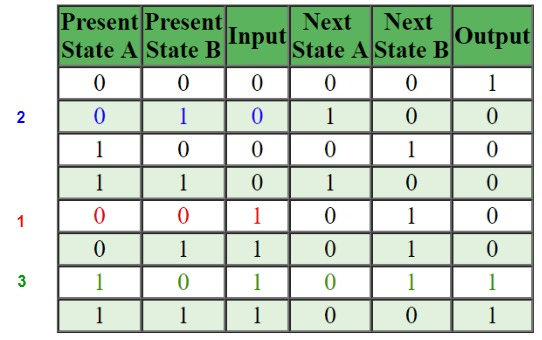# GATE | GATE-CS-2009 | Question 27

Given the following state table of an FSM with two states A and B, one input and one output:

 Present State A Present State B Input Next State A Next State B Output 0 0 0 0 0 1 0 1 0 1 0 0 1 0 0 0 1 0 1 1 0 1 0 0 0 0 1 0 1 0 0 1 1 0 0 1 1 0 1 0 1 1 1 1 1 0 0 1

If the initial state is A=0, B=0, what is the minimum length of an input string which will take the machine to the state A=0, B=1 with Output = 1?
(A) 3
(B) 4
(C) 5
(D) 6

Explanation: //(0, 0) –1–> (0, 1) –0–>(1, 0) –1–> (0, 1) and output 1

According to the question we have to reach the states A=0, B=1 and output=1. This state is shown by green color. Thus to reach final states as A=0, B=1 and output=1 we have to reach previous states of A=1, B=0. Since initial states are A=0,B=0 (red); we provide input=1 (to reach A=0,B=1) Now this will give present states as A=0, B=1 and output=0. Now we provide (blue) input=0 (to reach A=1,B=0) with present states as A=0, B=1.
The present states will become A=1, B=0 and output=0. This is what is required. On providing input=1 we get final states as A=0, B=1 and output=1.
Hence an input string of 3 i.e. 101 leads to the desired output and states.This solution is contributed by Kriti Kushwaha

Quiz of this Question

My Personal Notes arrow_drop_up
Article Tags :

Be the First to upvote.

Please write to us at contribute@geeksforgeeks.org to report any issue with the above content.